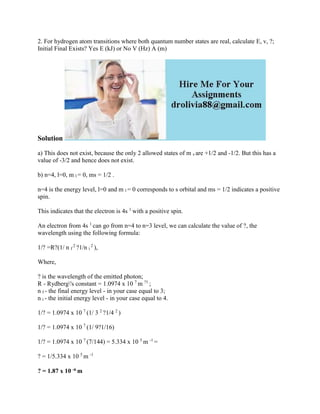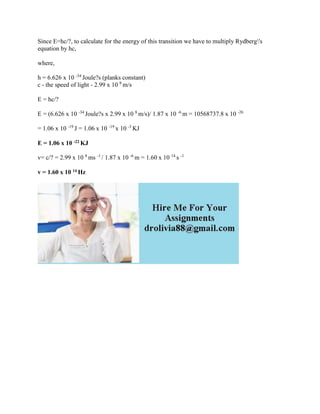Seu SlideShare está sendo baixado. ×

# 2- For hydrogen atom transitions where both quantum number states are.docx

Anúncio
Anúncio
Anúncio
Anúncio
Anúncio
Anúncio
Anúncio
Anúncio
Anúncio
Anúncio
AnúncioCarregando em…3
×

1 de 2 Anúncio

# 2- For hydrogen atom transitions where both quantum number states are.docx

2. For hydrogen atom transitions where both quantum number states are real, calculate E, v, ?; Initial Final Exists? Yes E (kJ) or No V (Hz) A (m)
Solution
a) This does not exist, because the only 2 allowed states of m s are +1/2 and -1/2. But this has a value of -3/2 and hence does not exist.
b) n=4, l=0, m l = 0, ms = 1/2 .
n=4 is the energy level, l=0 and m l = 0 corresponds to s orbital and ms = 1/2 indicates a positive spin.
This indicates that the electron is 4s 1 with a positive spin.
An electron from 4s 1 can go from n=4 to n=3 level, we can calculate the value of ?, the wavelength using the following formula:
1/? =R?(1/ n f 2 ?1/n i 2 ),
Where,
? is the wavelength of the emitted photon;
R - Rydberg\'s constant = 1.0974 x 10 7 m ?1 ;
n f - the final energy level - in your case equal to 3;
n i - the initial energy level - in your case equal to 4.
1/? = 1.0974 x 10 7 (1/ 3 2 ?1/4 2 )
1/? = 1.0974 x 10 7 (1/ 9?1/16)
1/? = 1.0974 x 10 7 (7/144) = 5.334 x 10 5 m -1 =
? = 1/5.334 x 10 5 m -1
? = 1.87 x 10 -6 m
Since E=hc/?, to calculate for the energy of this transition we have to multiply Rydberg\'s equation by hc,
where,
h = 6.626 x 10 -34 Joule?s (planks constant)
c - the speed of light - 2.99 x 10 8 m/s
E = hc/?
E = (6.626 x 10 -34 Joule?s x 2.99 x 10 8 m/s)/ 1.87 x 10 -6 m = 10568737.8 x 10 -26
= 1.06 x 10 -19 J = 1.06 x 10 -19 x 10 -3 KJ
E = 1.06 x 10 -22 KJ
v= c/? = 2.99 x 10 8 ms -1 / 1.87 x 10 -6 m = 1.60 x 10 14 s -1
v = 1.60 x 10 14 Hz
.

2. For hydrogen atom transitions where both quantum number states are real, calculate E, v, ?; Initial Final Exists? Yes E (kJ) or No V (Hz) A (m)
Solution
a) This does not exist, because the only 2 allowed states of m s are +1/2 and -1/2. But this has a value of -3/2 and hence does not exist.
b) n=4, l=0, m l = 0, ms = 1/2 .
n=4 is the energy level, l=0 and m l = 0 corresponds to s orbital and ms = 1/2 indicates a positive spin.
This indicates that the electron is 4s 1 with a positive spin.
An electron from 4s 1 can go from n=4 to n=3 level, we can calculate the value of ?, the wavelength using the following formula:
1/? =R?(1/ n f 2 ?1/n i 2 ),
Where,
? is the wavelength of the emitted photon;
R - Rydberg\'s constant = 1.0974 x 10 7 m ?1 ;
n f - the final energy level - in your case equal to 3;
n i - the initial energy level - in your case equal to 4.
1/? = 1.0974 x 10 7 (1/ 3 2 ?1/4 2 )
1/? = 1.0974 x 10 7 (1/ 9?1/16)
1/? = 1.0974 x 10 7 (7/144) = 5.334 x 10 5 m -1 =
? = 1/5.334 x 10 5 m -1
? = 1.87 x 10 -6 m
Since E=hc/?, to calculate for the energy of this transition we have to multiply Rydberg\'s equation by hc,
where,
h = 6.626 x 10 -34 Joule?s (planks constant)
c - the speed of light - 2.99 x 10 8 m/s
E = hc/?
E = (6.626 x 10 -34 Joule?s x 2.99 x 10 8 m/s)/ 1.87 x 10 -6 m = 10568737.8 x 10 -26
= 1.06 x 10 -19 J = 1.06 x 10 -19 x 10 -3 KJ
E = 1.06 x 10 -22 KJ
v= c/? = 2.99 x 10 8 ms -1 / 1.87 x 10 -6 m = 1.60 x 10 14 s -1
v = 1.60 x 10 14 Hz
.

Anúncio
Anúncio

### 2- For hydrogen atom transitions where both quantum number states are.docx

1. 1. 2. For hydrogen atom transitions where both quantum number states are real, calculate E, v, ?; Initial Final Exists? Yes E (kJ) or No V (Hz) A (m) Solution a) This does not exist, because the only 2 allowed states of m s are +1/2 and -1/2. But this has a value of -3/2 and hence does not exist. b) n=4, l=0, m l = 0, ms = 1/2 . n=4 is the energy level, l=0 and m l = 0 corresponds to s orbital and ms = 1/2 indicates a positive spin. This indicates that the electron is 4s 1 with a positive spin. An electron from 4s 1 can go from n=4 to n=3 level, we can calculate the value of ?, the wavelength using the following formula: 1/? =R?(1/ n f 2 ?1/n i 2 ), Where, ? is the wavelength of the emitted photon; R - Rydberg's constant = 1.0974 x 10 7 m ?1 ; n f - the final energy level - in your case equal to 3; n i - the initial energy level - in your case equal to 4. 1/? = 1.0974 x 10 7 (1/ 3 2 ?1/4 2 ) 1/? = 1.0974 x 10 7 (1/ 9?1/16) 1/? = 1.0974 x 10 7 (7/144) = 5.334 x 10 5 m -1 = ? = 1/5.334 x 10 5 m -1 ? = 1.87 x 10 -6 m
2. 2. Since E=hc/?, to calculate for the energy of this transition we have to multiply Rydberg's equation by hc, where, h = 6.626 x 10 -34 Joule?s (planks constant) c - the speed of light - 2.99 x 10 8 m/s E = hc/? E = (6.626 x 10 -34 Joule?s x 2.99 x 10 8 m/s)/ 1.87 x 10 -6 m = 10568737.8 x 10 -26 = 1.06 x 10 -19 J = 1.06 x 10 -19 x 10 -3 KJ E = 1.06 x 10 -22 KJ v= c/? = 2.99 x 10 8 ms -1 / 1.87 x 10 -6 m = 1.60 x 10 14 s -1 v = 1.60 x 10 14 Hz